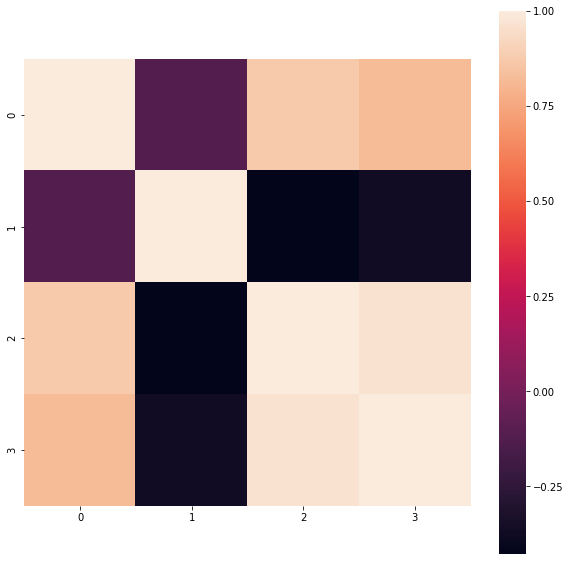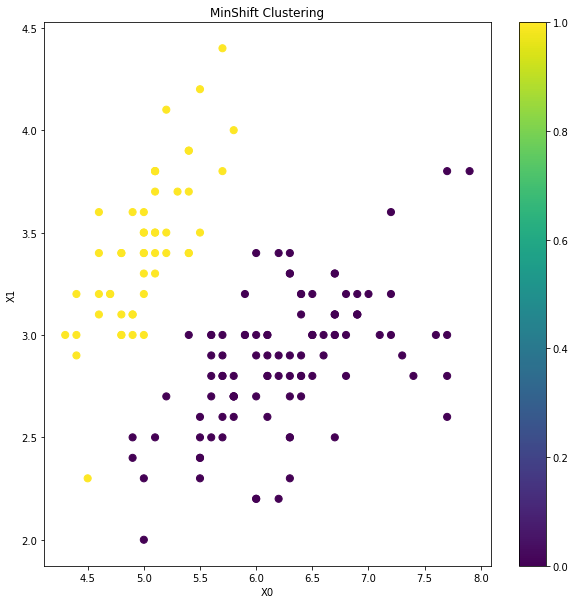How to do MinShift Clustering in Python?
MACHINE LEARNING RECIPES DATA CLEANING PYTHON DATA MUNGING PANDAS CHEATSHEET     ALL TAGS

# How to do MinShift Clustering in Python?

This recipe helps you do MinShift Clustering in Python

0
In :
```## How to do MinShift Clustering in Python
def Snippet_160():
print()
print(format('How to do MinShift based Clustering in Python','*^82'))

import warnings
warnings.filterwarnings("ignore")

from sklearn import datasets
from sklearn.preprocessing import StandardScaler
from sklearn.cluster import MeanShift
import pandas as pd
import seaborn as sns
import matplotlib.pyplot as plt

X = iris.data; data = pd.DataFrame(X)
cor = data.corr()

fig = plt.figure(figsize=(10,10))
sns.heatmap(cor, square = True); plt.show()

# Standarize features
scaler = StandardScaler()
X_std = scaler.fit_transform(X)

# Conduct MinShift Clustering
clt = MeanShift()

# Train model
model = clt.fit(X_std)

# Predict clusters
clusters = pd.DataFrame(model.fit_predict(X_std))
data['Cluster'] = clusters

# Visualise cluster membership
fig = plt.figure(figsize=(10,10)); ax = fig.add_subplot(111)
scatter = ax.scatter(data,data, c=data['Cluster'],s=50)
ax.set_title('MinShift Clustering')
ax.set_xlabel('X0'); ax.set_ylabel('X1')
plt.colorbar(scatter); plt.show()

Snippet_160()
```
```******************How to do MinShift based Clustering in Python*******************
```#### Relevant Projects

##### Predict Census Income using Deep Learning Models
In this project, we are going to work on Deep Learning using H2O to predict Census income.

##### Build an Image Classifier for Plant Species Identification
In this machine learning project, we will use binary leaf images and extracted features, including shape, margin, and texture to accurately identify plant species using different benchmark classification techniques.

##### Predict Churn for a Telecom company using Logistic Regression
Machine Learning Project in R- Predict the customer churn of telecom sector and find out the key drivers that lead to churn. Learn how the logistic regression model using R can be used to identify the customer churn in telecom dataset.

##### Data Science Project-TalkingData AdTracking Fraud Detection
Machine Learning Project in R-Detect fraudulent click traffic for mobile app ads using R data science programming language.

##### Learn to prepare data for your next machine learning project
Text data requires special preparation before you can start using it for any machine learning project.In this ML project, you will learn about applying Machine Learning models to create classifiers and learn how to make sense of textual data.

##### Mercari Price Suggestion Challenge Data Science Project
Data Science Project in Python- Build a machine learning algorithm that automatically suggests the right product prices.

##### Credit Card Fraud Detection as a Classification Problem
In this data science project, we will predict the credit card fraud in the transactional dataset using some of the predictive models.

##### Solving Multiple Classification use cases Using H2O
In this project, we are going to talk about H2O and functionality in terms of building Machine Learning models.

##### Identifying Product Bundles from Sales Data Using R Language
In this data science project in R, we are going to talk about subjective segmentation which is a clustering technique to find out product bundles in sales data.

##### Ecommerce product reviews - Pairwise ranking and sentiment analysis
This project analyzes a dataset containing ecommerce product reviews. The goal is to use machine learning models to perform sentiment analysis on product reviews and rank them based on relevance. Reviews play a key role in product recommendation systems.